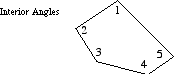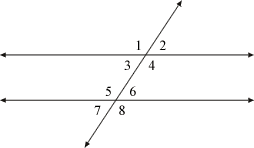# interior angle

Interior Angle

An angle on the interior of a plane figure.

Examples: The angles labeled 1, 2, 3, 4, and 5 in the pentagon below are all interior angles. Angles 3, 4, 5, and 6 in the second example below are all interior angles as well (parallel lines cut by a transversal).

Note: The sum of the interior angles of an n-gon is given by the formula (n – 2)·180°. For a triangle this sum is 180°, a quadrilateral 360°, a pentagon 540°, etc.Parallel lines cut by a transversal